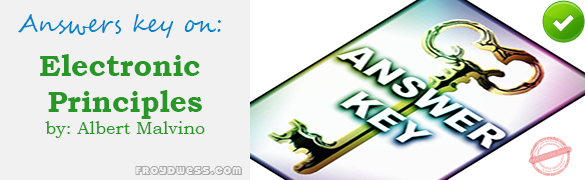# MCQs in Transistor Biasing – Answers

(Last Updated On: December 8, 2017)Below are the answers key for Multiple Choice Questions in Chapter 8: Transistor Biasing from the book Electronic Principles 7th Edition by Albert Malvino. Make sure to familiarize each and every questions to increase the chance of passing the ECE Board Exam.

1. d. Ground

2. a. Base voltage

3. a. Less than the base supply voltage

4. d. Stable Q point

5. d. Decrease the emitter current

6. b. Emitter bias

7. b. Only one supply

8. a. Active region

9. c. Current gain

10. c. Increases

11. b. Switching circuits

12. b. Drop in half

13. a. Decrease

14. c. Almost totally insensitive to changes in current gain

15. c. Near 0 V

16. a. Drop in half

17. b. Equal the collector supply voltage

18. C. Increase

19. d. Equal the collector supply voltage

20. a. Small

21. c. Current gain

22. a. Holes

23. d. The ratio of collector current to base current

24. b. Emitter current

25. b. Opposite npn currents

26. c. Resistors

Rate this: